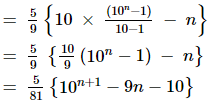Find the sum of the series 5 + 55 + 555 + … to n terms.

Asked by Sakshi | 1 year ago |  73

##### Solution :-

5 + 55 + 555 + … to n terms.

Let us take 5 as a common term so we get,

5 [1 + 11 + 111 + … n terms]

Now multiply and divide by 9 we get,

$$\dfrac{5}{9}$$ [9 + 99 + 999 + … n terms]

$$\dfrac{5}{9}$$[(10 – 1) + (102 – 1) + (103 – 1) + … n terms]

$$\dfrac{5}{9}$$[(10 + 102 + 103 + … n terms) – n]

So the G.P is

$$\dfrac{5}{9}$$[(10 + 102 + 103 + … n terms) – n]

Where, a = 10, r = $$\dfrac{10^2}{10}$$ = 10, n = nAnswered by Sakshi | 1 year ago

### Related Questions

#### Construct a quadratic in x such that A.M. of its roots is A and G.M. is G.

Construct a quadratic in x such that A.M. of its roots is A and G.M. is G.

#### Find the two numbers whose A.M. is 25 and GM is 20.

Find the two numbers whose A.M. is 25 and GM is 20.

#### If a is the G.M. of 2 and 1/4 find a.

If a is the G.M. of 2 and $$\dfrac{1}{4}$$ find a.

#### Find the geometric means of the following pairs of numbers

Find the geometric means of the following pairs of numbers:

(i) 2 and 8

(ii) a3b and ab3

(iii) –8 and –2

Insert 5 geometric means between $$\dfrac{32}{9}$$ and $$\dfrac{81}{2}$$.